## 9th Class Science Notes in English chapter 10 Gravitation

CBSE Revision Notes for CBSE Class 09 Science Gravitation Gravitation: Gravitation; Universal Law of Gravitation, Force of Gravitation of the earth (gravity), Acceleration due to Gravity; Mass and Weight; Free fall.

# 👉 GRAVITATION 👈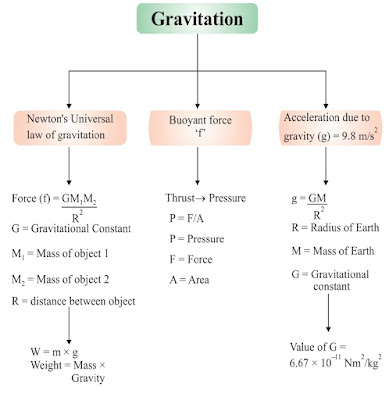## ✳️Gravitation:-

🔹 Earth attracts everything towards it by an unseen force of attraction. This force of attraction is known as gravitation or gravitational pull.

## ✳️Universal Law of Gravitation:-

🔹 Every object in the universe attracts other object by a force of attraction, called gravitation, which is directly proportional to the product of masses of the objects and inversely proportional to the square of distance between them.

🔹 Let masses (M) and (m) of two objects are distance (d) apart, then force of attraction (F) between them.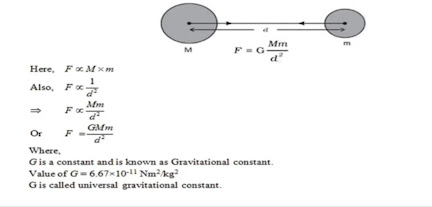## ✳️Relation between Newton’s third law of motion and Newton's law of gravitation:-

🔹 According to Newton's third law of motion, "Every object exerts equal and opposite force on other object but in opposite direction."

🔹 According to Newton's law of gravitation, “Every mass in the universe attracts the every other mass."

🔹 In case of freely falling stone and earth, stone is attracted towards earth means earth attracts the stone but according to Newton's third law of motion, the stone should also attract the earth and really it is true that stone also attracts the earth with the same force F = m x a.

🔹 but due to very less mass of the stone, the acceleration (a) in its velocity is 9.8 m/s² and acceleration (a) of earth towards stone is 1.65 x 10-24 m/s² which is negligible and we cannot feel it.

## ✳️Importance of Universal Law of Gravitation:-

🔹 It binds us to the earth.
🔹 It is responsible for the motion of the moon around the earth.
🔹 It is responsible for the motion of planets around the Sun.
🔹 Gravitational force of moon causes tides in seas on earth.

## ✳️Free fall of an object and acceleration during free fall:-

🔹 When an object is thrown upward, it reaches certain height, then it starts falling down towards earth. It is because the earth's gravitational force exerts on it.
🔹 This fall under the influence of earth is called 'free fall of an object'.
🔹 During this free fall direction do not change but velocity continuously changes which is called acceleration due to gravity.
🔹 It is denoted by 'g'. Its unit is same as acceleration m/s².

## ✳️Relationship and difference between 'G’ and ʻg:-

🔹 G = Gravitational constant
🔹 g = Acceleration due to gravity
🔹 g = GM / R²

## ✳️Difference between 'G’ and g"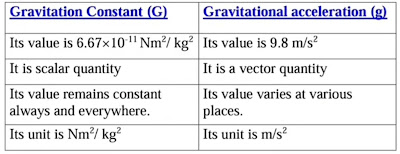## ✳️Difference between Mass and Weight :-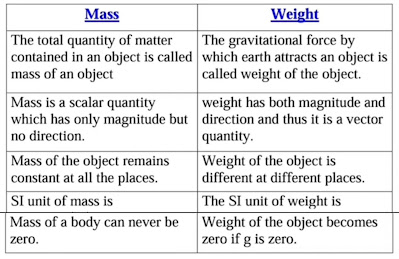## ✳️Factors affecting value of ʻg’ :-

🔹 Earth is not a perfect sphere. The radius of earth increases when we go from pole to equator. Therefore, in most of the calculation, we can take g as constant at the surface of earth or closer to it.

## ✴️ Pressure :-

🔹 The effect of thrust per unit area is called pressure
🔹 Pressure (P) = Force (F) Area (A) SI unit of pressure is Pascal denoted by 'Pa' or N/m²

### 👉 Pressure depends on two factors:-

🔹 (i) Force applied
🔹 (ii) Area of surface over which force

#### ▶ Examples:-

🔹 1. The base of high buildings is made wider so that weight of walls act over a large surface area and pressure is less.

🔹 2. School bags are having broad strap so that the weight of school bags fall over a larger area of the shoulder and produce less pressure and becomes less painful.

🔹 3. The blades of knives are made sharp so very small surface area and on applying force, it produces large pressure and cuts the object easily.

## ✳️ Buoyancy:-

🔹 The upward force experienced by an object when it is immersed into a fluid is called force of buoyancy. It acts in upward direction and it depends on the density of the fluid.

🔹 Force of gravitational attraction of the earth on the surface of the object < buoyant force exerted by fluid on the surface of the object.

#### 👉 Result:- The object floats

🔹 Force of gravitational attraction of the earth on the surface of the object > buoyant force exerted by fluid on the surface of the object.

#### 👉Result:- The object sinks

🔹 That is why, allpin sinks and boat/ship floats on the surface of water. (Archimedes' principle)

## ✳️ Density:-

🔹 The mass per unit volume is called density of an object. If M is the mass and V is the volume, then density (d) is▶ SI unit = kg/m³

## ✳️ Archimedes’ Principle :-

🔹 It states, when a body is immersed fully or partially in a fluid, it experiences a upward force that is equal to the weight of the fluid displaced by it.

### ✴️ Applications of Archimedes’ Principle :-

🔹 1) It is used in determining relative density of substances
🔹 2) It in designing ships and submarines.
🔹 3) Hydrometers and lactometers are made on this principle

🔹 It is because of this ship made of iron and steel floats in water whereas a small piece of iron sinks in it.

## ✳️ Relative density :-

🔹 The ratio of the density of a substance to that of the density of water is called relative density.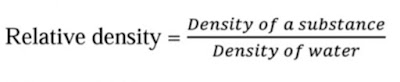It has no SI Unit.